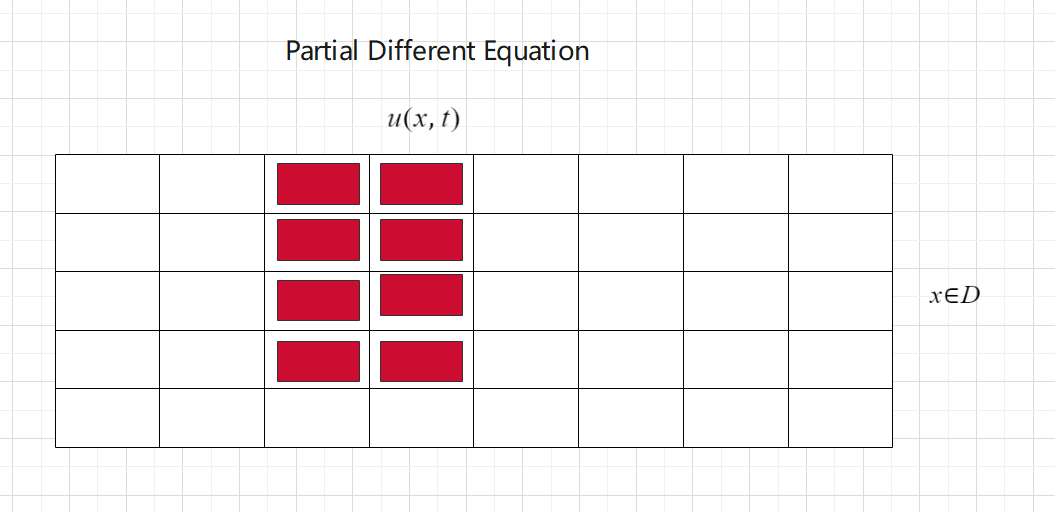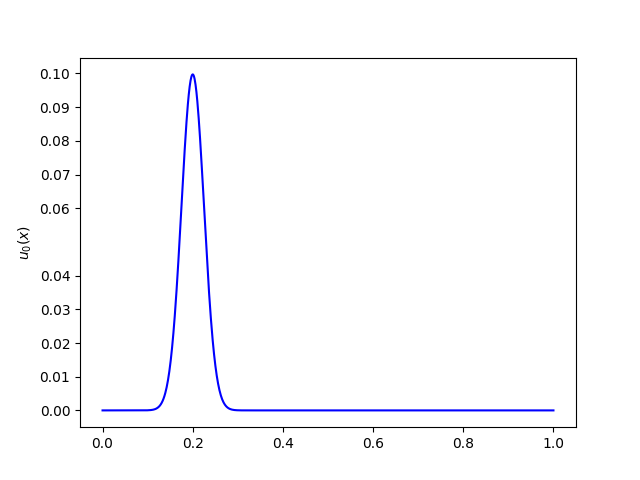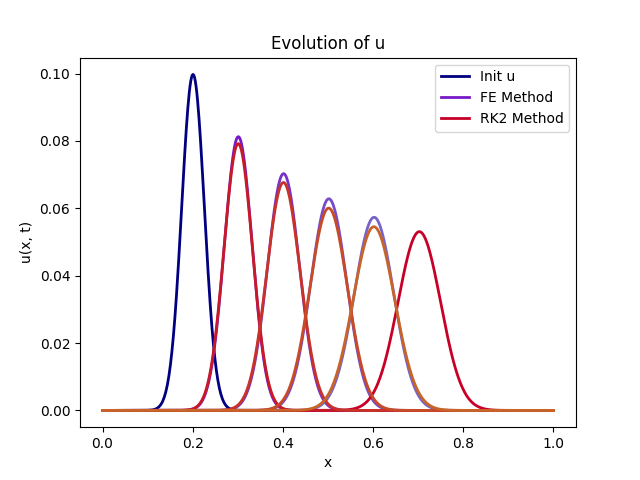# 偏微分方程

• 学的时候记得笔记都是英语的加上又懒得翻译就只好拖到了现在

## General

• 偏微分方程（PDE, partial differential equation）的解是一个关于时间和空间的方程 $u(x, t)$，我们用 u 代表在特定时间和空间的函数值。
• 在常微分方程的数值解中，我们是通过将 0-T 划分为若干个 $\Delta t$，通过计算每个 $\Delta t$ 位置的值来计算 $u(t)$。在偏微分方程中，我们构造下面的图形（mesh）来辅助我们的计算：• 有限元法（Finite Element Methods）
• 有限差分法（Finite Difference Methods）
• 有限体积法（Finite Volume Methods）

## Finite Difference Formulas

k 代表导热系数。

• 一阶偏导的前向差分(Forward difference for first spatial derivative)
• 一阶偏导的向后差分(Backward difference for first spatial derivative)
• 一阶偏导的中心差分(Central difference for first spatial derivative)
• 二阶偏导的中心差分(Central difference for second spatial derivative)

## Discretization Stencil

• 我们在网格来近似计算空间函数$u(x, t)$偏导（前向差分，中心差分等等）的这种方法叫做stencil，如果用空间内更多更远的点来近似一个点的偏导值，那么一般情况下误差就会变的更小。但对于有些情况却不同，补充的来说，用更多的点近似来减少误差只对光滑的函数有效，对于那些不连续的函数，相邻点之间的关系很小，使用太多的点反而会影响准确度。
• 我们用 stencils size 来描述有多少个点被用于近似偏导值。我们的误差和使用的近似方法的阶数成正比关系。我们假设误差为 e，h 为 网格缩小比例，q 是使用方法的阶数，我们有这样的关系：$e \sim h^q$
• 需要注意：减小空间网格的大小的同时也要减小每一步的时间$\Delta t$来确保稳定性。

## Solving PDE

• 解决 PDE 问题就是解决一个初始边界值问题（initial boundary value problem）：$u(x, t)$ 在给定的初始条件和边界条件下是如何变化的。

f包含边界条件的信息。A 是一个 $N-1 \times N-1$ 的系数矩阵，当x=1和x=N-1的时候都无法计算，所以设置行列式为全 0，需要 f 来辅助确定值以保证边界条件：

We discretize the spatial domain into N spaces with N+1 grid points. How many unknown variables are evolved in the resulting system of ODEs?

## Boundary Conditions

• Dirichlet conditions

Dirichlet conditions fix the value of the solution u(x,t) at the boundary. This is like pinning the solution to a certain value at the boundary.

• Neumann conditions

Neumann conditions fix the value, not of the solution, but of its spatial derivative $\frac{\partial u}{\partial x}$ at the boundary. This is like fixing the stresses at the boundary.

• Robin conditions

Robin conditions fix the value of a linear combination of the solution itself and its partial derivative at the boundary.

## Linear System

• A 是一般矩阵（general）：高斯消元（Gaussian elimination），$O(N^3)$
• A 是三角矩阵（triangular）：Forward substitution/backward substitution，$O(N^2)$
• A 是三对角线矩阵（tri-diagonal）：托马斯算法（Thomas algorithm），$O(N)$

## SampleFE 误差为 8% 左右，如果我们使用 RK2 代替 FE 方法，误差减小到 0.8%。（用的黑盒函数测的误差，暂时还没复现解析解）

Ctwo

2021-03-02

2021-03-04